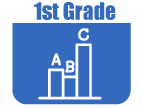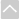OER Curriculum

These Open Educational Resources are comprehensive and coherent curricular materials that may be used to teach a course or grade level.

Engage NY
• Grade 1 Math Module 1: Sums and Differences to 10 (EngageNY)
In this first module of Grade 1, students make significant progress towards fluency with addition and subtraction of numbers to 10 as they are presented with opportunities intended to advance them from counting all to counting on which leads many students then to decomposing and composing addends and total amounts.
• Grade 1 Math Module 2: Intro to Place Value Through Addition and Subtraction Within 20 (EngageNY)
Module 2 serves as a bridge from students' prior work with problem solving within 10 to work within 100 as students begin to solve addition and subtraction problems involving teen numbers. Students go beyond the Level 2 strategies of counting on and counting back as they learn Level 3 strategies informally called "make ten" or "take from ten."
• Grade 1 Math Module 3: Ordering and Comparing Length Measurements as Numbers (EngageNY)
Module 3 begins by extending students' kindergarten experiences with direct length comparison to indirect comparison whereby the length of one object is used to compare the lengths of two other objects. Longer than and shorter than are taken to a new level of precision by introducing the idea of a length unit. Students then explore the usefulness of measuring with similar units. The module closes with students representing and interpreting data.
• Grade 1 Math Module 4: Place Value, Comparison, Addition and Subtraction to 40 (EngageNY)
Module 4 builds upon Module 2's work with place value within 20, now focusing on the role of place value in the addition and subtraction of numbers to 40. Students study, organize, and manipulate numbers within 40. They compare quantities and begin using the symbols for greater than (>) and less than (<). Addition and subtraction of tens is another focus of this module as is the use of familiar strategies to add two-digit and single-digit numbers within 40. Near the end of the module, the focus moves to new ways to represent larger quantities and adding like place value units as students add two-digit numbers.
• Grade 1 Math Module 5: Identifying, Composing, and Partitioning Shapes (EngageNY)
In Module 5, students consider part-whole relationships through a geometric lens. The module opens with students identifying the defining parts, or attributes, of two- and three-dimensional shapes, building on their kindergarten experiences of sorting, analyzing, comparing, and creating various two- and three-dimensional shapes and objects. Students combine shapes to create a new whole: a composite shape. They also relate geometric figures to equal parts and name the parts as halves and fourths. The module closes with students applying their understanding of halves to tell time to the hour and half hour.
• Grade 1 Math Module 6: Place Value, Comparison, Addition and Subtraction to 100 (EngageNY)
In this final module of the Grade 1 curriculum, students bring together their learning from Module 1 through Module 5 to learn the most challenging Grade 1 standards and celebrate their progress. As the module opens, students grapple with comparative word problem types. Next, they extend their understanding of and skill with tens and ones to numbers to 100. Students also extend their learning from Module 4 to the numbers to 100 to add and subtract. At the start of the second half of Module 6, students are introduced to nickels and quarters, having already used pennies and dimes in the context of their work with numbers to 40 in Module 4. Students use their knowledge of tens and ones to explore decompositions of the values of coins. The module concludes with fun fluency festivities to celebrate a year's worth of learning.
•Georgia Standards of Excellence Curriculum Frameworks
• Georgia Standards of Excellence Mathematics
GeorgiaStandards.Org (GSO) is a free, public website providing information and resources necessary to help meet the educational needs of students. The goal of this web site is to provide information that will enhance and support teaching and learning of Georgia standards.
• Grade 1 Unit 1: Creating Routines Using Data (Georgia Standards)
In this unit, students will establish daily math routines to be carried out throughout the year, such as lunch count, daily questions, calendar activities, working with a 0-99 chart, etc.
• Grade 1 Unit 2: Developing Base Ten Number Sense (Georgia Standards)
In this unit, students will rote count forward to 120 by counting on from any number less than 120, represent a quantity using numerals, locate 0-100 on a number line, use the strategies of counting on and counting back to understand number relationships and explore with the 99 chart to see patterns between numbers, such as, all of the numbers in a column on the hundreds chart have the same digit in the ones place, and all of the numbers in a row have the same digit in the tens place.
• Grade 1 Unit 3: Operations and Algebraic Thinking (Georgia Standards)
In this unit, students will explore, understand, and apply the commutative and associative properties as strategies for solving addition problems. Share, discuss, and compare strategies as a class. Connect counting on to solving subtraction problems. For the problem 15 7 = ? they think about the number they have to count on from 7 to get to 15. Work with sums and differences less than or equal to 20 using the numbers 0 to 20. Identify and then apply a pattern or structure in mathematics. For example, pose a string of addition and subtraction problems involving the same three numbers chosen from the numbers 0 to 20, such as 4 + 13 = 17 and 13 + 4 = 17. Analyze number patterns and create conjectures or guesses.
• Grade 1 Unit 4: Sorting, Comparing, and Ordering (Georgia Standards)
In this unit students will develop an understanding of linear measurement, measure lengths as iterating length units, tell and write time to the hour and half hour and represent and interpret data.
• Grade 1 Unit 5: Understanding Place Value (Georgia Standards)
In this unit, students will understand the order of the counting numbers and their relative magnitudes, use a number line and 99 chart to build understanding of numbers and their relation to other numbers, unitize a group of ten ones as a whole unit: a ten, and understand that a group of ten pennies is equivalent to a dime.
• Grade 1 Unit 6: Understanding Shapes and Fractions (Georgia Standards)
In this unit, students will study and compose two- and three-dimensional figures, identify basic figures within two- and three-dimensional figures, compare, contrast, and/or classify geometric shapes using position, shape, size, number of sides, and number of angles, solve simple problems, including those involving spatial relationships, investigate and predict the results of putting together and taking apart two- and three-dimensional shapes, create mental images of geometric shapes using spatial memory and spatial visualization, relate, identify, partition, and label fractions (halves, fourths) as equal parts of whole objects and apply terms such as half of, quarter of, to describe equal shares.
•The Online Core Resource pages are a collaborative project between the Utah State Board of Education and the Utah Education Network. If you would like to recommend a high quality resource, contact Trish French (Elementary) or Lindsey Henderson (Secondary). If you find inaccuracies or broken links contact resources@uen.org.